• +91 9971497814
• info@interviewmaterial.com

# Chapter 15 Statistics Ex-15.2 Interview Questions Answers

### Related Subjects

Question 1 : Find the mean and variance for the data
6, 7, 10, 12, 13, 4, 8, 12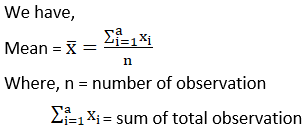So, x̅ = (6 + 7 + 10 + 12 + 13 + 4 + 8 + 12)/8
= 72/8
= 9
Let us make the table of the given data and append other columns after calculations.
 Xi Deviations from mean (xi – x̅) (xi – x̅)2 6 6 – 9 = -3 9 7 7 – 9 = -2 4 10 10 – 9 = 1 1 12 12 – 9 = 3 9 13 13 – 9 = 4 16 4 4 – 9 = – 5 25 8 8 – 9 = – 1 1 12 12 – 9 = 3 9 74

We know that Variance,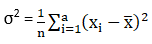σ2 =(1/8) × 74

= 9.2

Mean = 9 and Variance= 9.25

Question 2 : Find the mean and variance for the data

First n natural numbers

We know that Mean =Sum of all observations/Number of observations

Mean, x̅ = ((n(n +1))2)/n

= (n + 1)/2

and also WKT Variance,By substitute thatvalue of x̅ we get,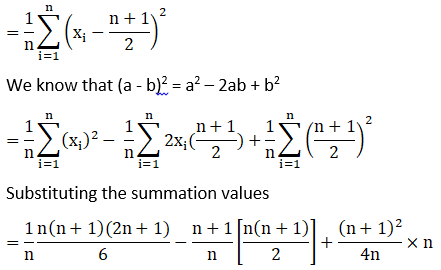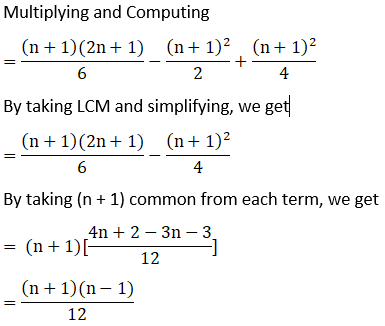WKT, (a + b)(a – b) =a2 – b2

σ2 =(n2 – 1)/12

Mean = (n + 1)/2 andVariance = (n2 – 1)/12

Question 3 : Find the mean and variance for the data
First 10 multiples of 3

First we have to writethe first 10 multiples of 3,

3, 6, 9, 12, 15, 18,21, 24, 27, 30So, x̅ = (3 + 6 + 9 +12 + 15 + 18 + 21 + 24 + 27 + 30)/10

= 165/10

= 16.5

Let us make the tableof the data and append other columns after calculations.

 Xi Deviations from mean (xi – x̅) (xi – x̅)2 3 3 – 16.5 = -13.5 182.25 6 6 – 16.5 = -10.5 110.25 9 9 – 16.5 = -7.5 56.25 12 12 – 16.5 = -4.5 20.25 15 15 – 16.5 = -1.5 2.25 18 18 – 16.5 = 1.5 2.25 21 21 – 16.5 = – 4.5 20.25 24 24 – 16.5 = 7.5 56.25 27 27 – 16.5 = 10.5 110.25 30 30 – 16.5 = 13.5 182.25 742.5

Then, Variance= (1/10) × 742.5

= 74.25

Mean = 16.5 andVariance = 74.25

Question 4 : Find the mean and variance for the data
 xi 6 10 14 18 24 28 30 fi 2 4 7 12 8 4 3

Let us make the tableof the given data and append other columns after calculations.

 Xi fi fixi Deviations from mean (xi – x̅) (xi – x̅)2 fi(xi – x̅)2 6 2 12 6 – 19 = 13 169 338 10 4 40 10 – 19 = -9 81 324 14 7 98 14 – 19 = -5 25 175 18 12 216 18 – 19 = -1 1 12 24 8 192 24 – 19 = 5 25 200 28 4 112 28 – 19 = 9 81 324 30 3 90 30 – 19 = 11 121 363 N = 40 760 1736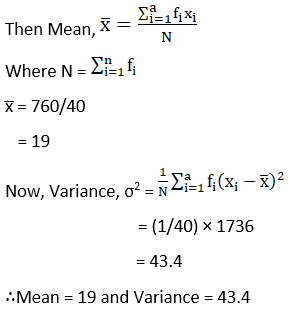Question 5 : Find the mean and variance for the data
 xi 92 93 97 98 102 104 109 fi 3 2 3 2 6 3 3

Let us make the tableof the given data and append other columns after calculations.

 Xi fi fixi Deviations from mean (xi – x̅) (xi – x̅)2 fi(xi – x̅)2 92 3 276 92 – 100 = -8 64 192 93 2 186 93 – 100 = -7 49 98 97 3 291 97 – 100 = -3 9 27 98 2 196 98 – 100 = -2 4 8 102 6 612 102 – 100 = 2 4 24 104 3 312 104 – 100 = 4 16 48 109 3 327 109 – 100 = 9 81 243 N = 22 2200 640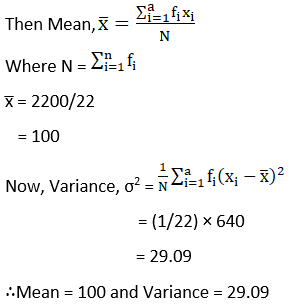Question 6 : Find the mean and standard deviation using short-cut method.
 xi 60 61 62 63 64 65 66 67 68 fi 2 1 12 29 25 12 10 4 5

Let the assumed mean A= 64. Here h = 1

We obtain thefollowing table from the given data.

 Xi Frequency fi Yi = (xi – A)/h Yi2 fiyi fiyi2 60 2 -4 16 -8 32 61 1 -3 9 -3 9 62 12 -2 4 -24 48 63 29 -1 1 -29 29 64 25 0 0 0 0 65 12 1 1 12 12 66 10 2 4 20 40 67 4 3 9 12 36 68 5 4 16 20 80 0 286

Mean,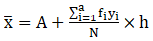Where A = 64, h = 1

So, x̅ = 64 + ((0/100)× 1)

= 64 + 0

= 64

Then, variance,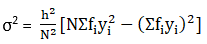σ2 =(12/1002) [100(286) – 02]

= (1/10000) [28600 –0]

= 28600/10000

= 2.86

Hence, standarddeviation = σ = √2.886

= 1.691

Mean = 64 andStandard Deviation = 1.691

Question 7 : Find the mean and variance for the following frequency distributions in Exercises 7 and 8.
 Classes 0 – 30 30 – 60 60 – 90 90 – 120 120 – 150 150 – 180 180 – 210 Frequencies 2 3 5 10 3 5 2

Let us make the tableof the given data and append other columns after calculations.

 Classes Frequency fi Mid – points xi fixi (xi – x̅) (xi – x̅)2 fi(xi – x̅)2 0 – 30 2 15 30 -92 8464 16928 30 – 60 3 45 135 -62 3844 11532 60 – 90 5 75 375 -32 1024 5120 90 – 120 10 105 1050 -2 4 40 120 – 150 3 135 405 28 784 2352 150 – 180 5 165 825 58 3364 16820 180 – 210 2 195 390 88 7744 15488 N = 30 3210 68280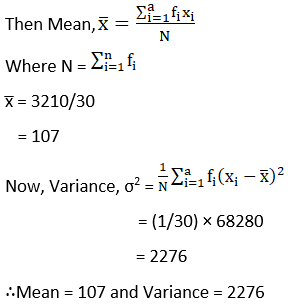Question 8 : Find the mean and variance for the following frequency distributions in Exercises 7 and 8.
 Classes 0 – 10 10 – 20 20 – 30 30 – 40 40 –50 Frequencies 5 8 15 16 6

Let us make the tableof the given data and append other columns after calculations.

 Classes Frequency fi Mid – points xi fixi (xi – x̅) (xi – x̅)2 fi(xi – x̅)2 0 – 10 5 5 25 -22 484 2420 10 – 20 8 15 120 -12 144 1152 20 – 30 15 25 375 -2 4 60 30 – 40 16 35 560 8 64 1024 40 –50 6 45 270 18 324 1944 N = 50 1350 6600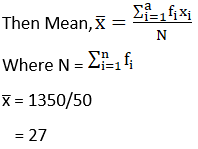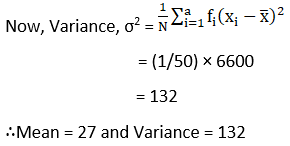Question 9 : Find the mean, variance and standard deviation using short-cut method
 Height in cms 70 – 75 75 – 80 80 – 85 85 – 90 90 – 95 95 – 100 100 – 105 105 – 110 110 – 115 Frequencies 3 4 7 7 15 9 6 6 3

Let the assumed mean,A = 92.5 and h = 5

Let us make the tableof the given data and append other columns after calculations.

 Height (class) Number of children Frequency fi Midpoint Xi Yi = (xi – A)/h Yi2 fiyi fiyi2 70 – 75 2 72.5 -4 16 -12 48 75 – 80 1 77.5 -3 9 -12 36 80 – 85 12 82.5 -2 4 -14 28 85 – 90 29 87.5 -1 1 -7 7 90 – 95 25 92.5 0 0 0 0 95 – 100 12 97.5 1 1 9 9 100 – 105 10 102.5 2 4 12 24 105 – 110 4 107.5 3 9 18 54 110 – 115 5 112.5 4 16 12 48 N = 60 6 254

Mean,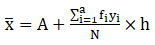Where, A = 92.5, h = 5

So, x̅ = 92.5 +((6/60) × 5)

= 92.5 + ½

= 92.5 + 0.5

= 93

Then, Variance,σ2 =(52/602) [60(254) – 62]

= (1/144) [15240 – 36]

= 15204/144

= 1267/12

= 105.583

Hence, standarddeviation = σ = √105.583

= 10.275

Mean = 93,variance = 105.583 and Standard Deviation = 10.275

Question 10 :
The diameters of circles (in mm) drawn in a design are given below:
 Diameters 33 – 36 37 – 40 41 – 44 45 – 48 49 – 52 No. of circles 15 17 21 22 25
Calculate the standard deviation and mean diameter of the circles.
[Hint first make the data continuous by making the classes as 32.5-36.5, 36.5-40.5, 40.5-44.5, 44.5 – 48.5, 48.5 – 52.5 and then proceed.]

Let the assumed mean,A = 42.5 and h = 4

Let us make the tableof the given data and append other columns after calculations.

 Height (class) Number of children (Frequency fi) Midpoint Xi Yi = (xi – A)/h Yi2 fiyi fiyi2 32.5 – 36.5 15 34.5 -2 4 -30 60 36.5 – 40.5 17 38.5 -1 1 -17 17 40.5 – 44.5 21 42.5 0 0 0 0 44.5 – 48.5 22 46.5 1 1 22 22 48.5 – 52.5 25 50.5 2 4 50 100 N = 100 25 199

Mean,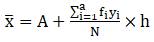Where, A = 42.5, h = 4

So, x̅ = 42.5 +(25/100) × 4

= 42.5 + 1

= 43.5

Then, Variance,σ2 =(42/1002)[100(199) – 252]

= (1/625) [19900 –625]

= 19275/625

= 771/25

= 30.84

Hence, standarddeviation = σ = √30.84

= 5.553

Mean = 43.5,variance = 30.84 and Standard Deviation = 5.553.

Todays Deals### Chapter 15 Statistics Ex-15.2 Contributorskrishan

Name:
Email:

# Latest News# 9000 interview questions in different categories